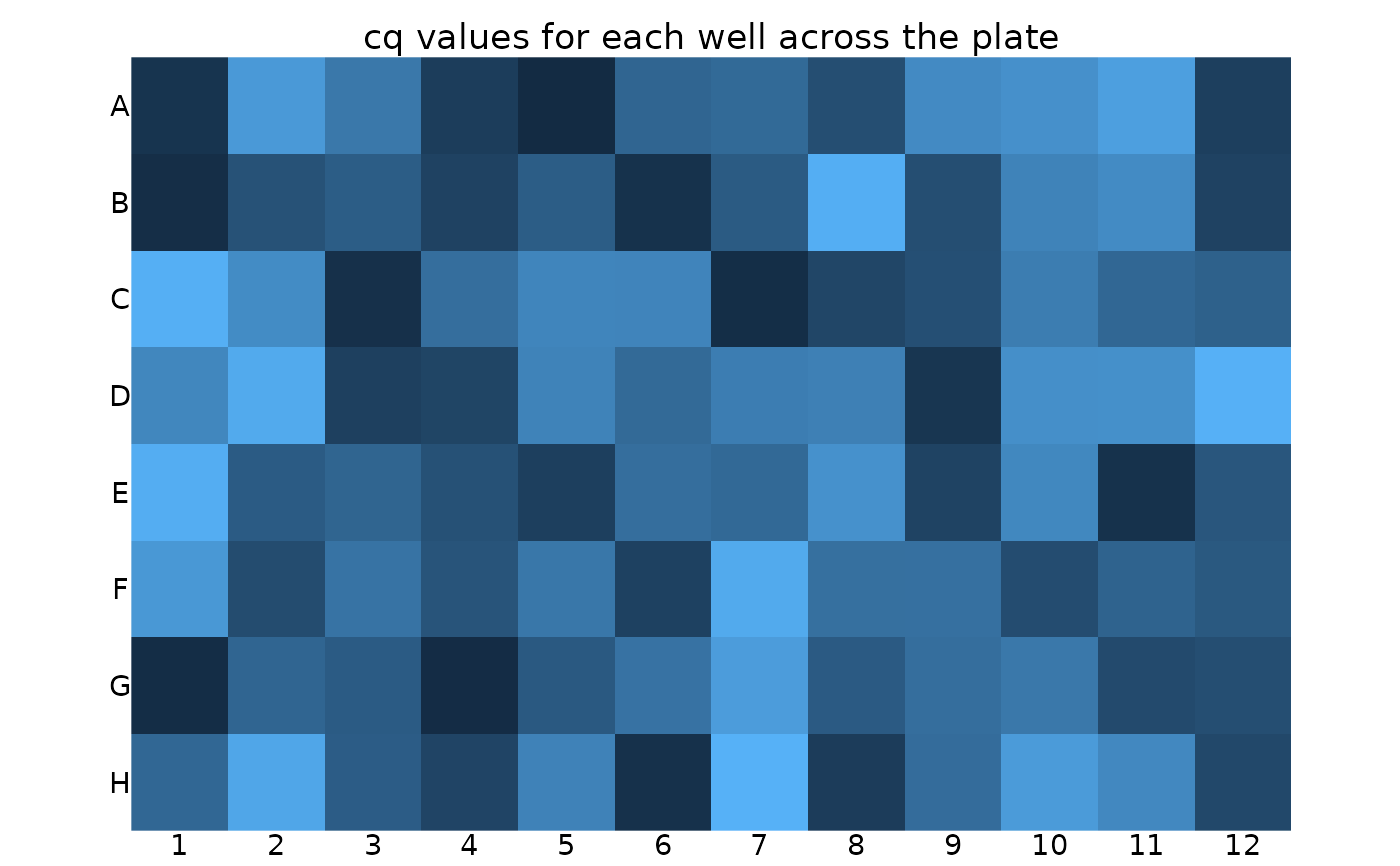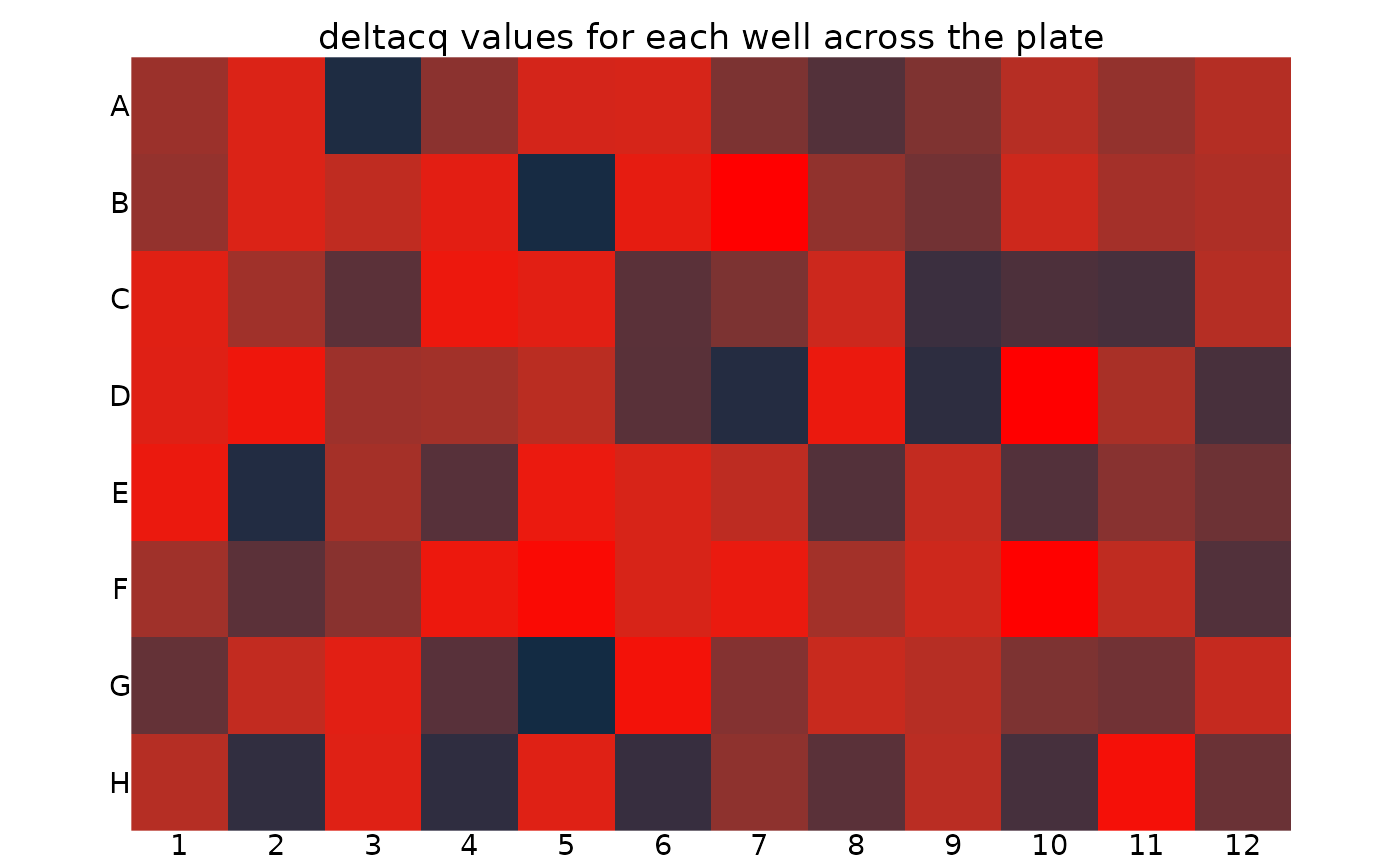Plots the plate with each well coloured by its value. Example values are Cq, Delta Cq or Delta Delta Cq.

## Usage

``display_plate_value(plate, value = "cq")``

## Arguments

plate

tibble with variables well_col, well_row, and the variable to be plotted.

value

character vector selecting the variable in plate to plot as the well value

## Value

ggplot object; major output is to plot it

## Details

For a specific example see the calibration vignette: `vignette("calibration_vignette", package = "tidyqpcr")`

Other plate creation functions: `create_blank_plate()`, `create_colkey_4diln_2ctrl_in_24()`, `create_colkey_6_in_24()`, `create_colkey_6diln_2ctrl_in_24()`, `create_rowkey_4_in_16()`, `create_rowkey_8_in_16_plain()`, `display_plate_qpcr()`, `display_plate()`, `label_plate_rowcol()`, `make_row_names_echo1536()`, `make_row_names_lc1536()`

## Examples

``````library(dplyr)
#>
#> Attaching package: ‘dplyr’
#> The following objects are masked from ‘package:stats’:
#>
#>     filter, lag
#> The following objects are masked from ‘package:base’:
#>
#>     intersect, setdiff, setequal, union
library(ggplot2)

# create 96 well plate with random values
plate_randomcq <- create_blank_plate_96well() %>%
mutate(cq = runif(96) * 10,
deltacq = runif(96) * 2)

# display well Cq value across plate
display_plate_value(plate_randomcq)# display well Delta Cq value across plate with red colour pallette
display_plate_value(plate_randomcq, value = "deltacq") +   # uses ggplot syntax